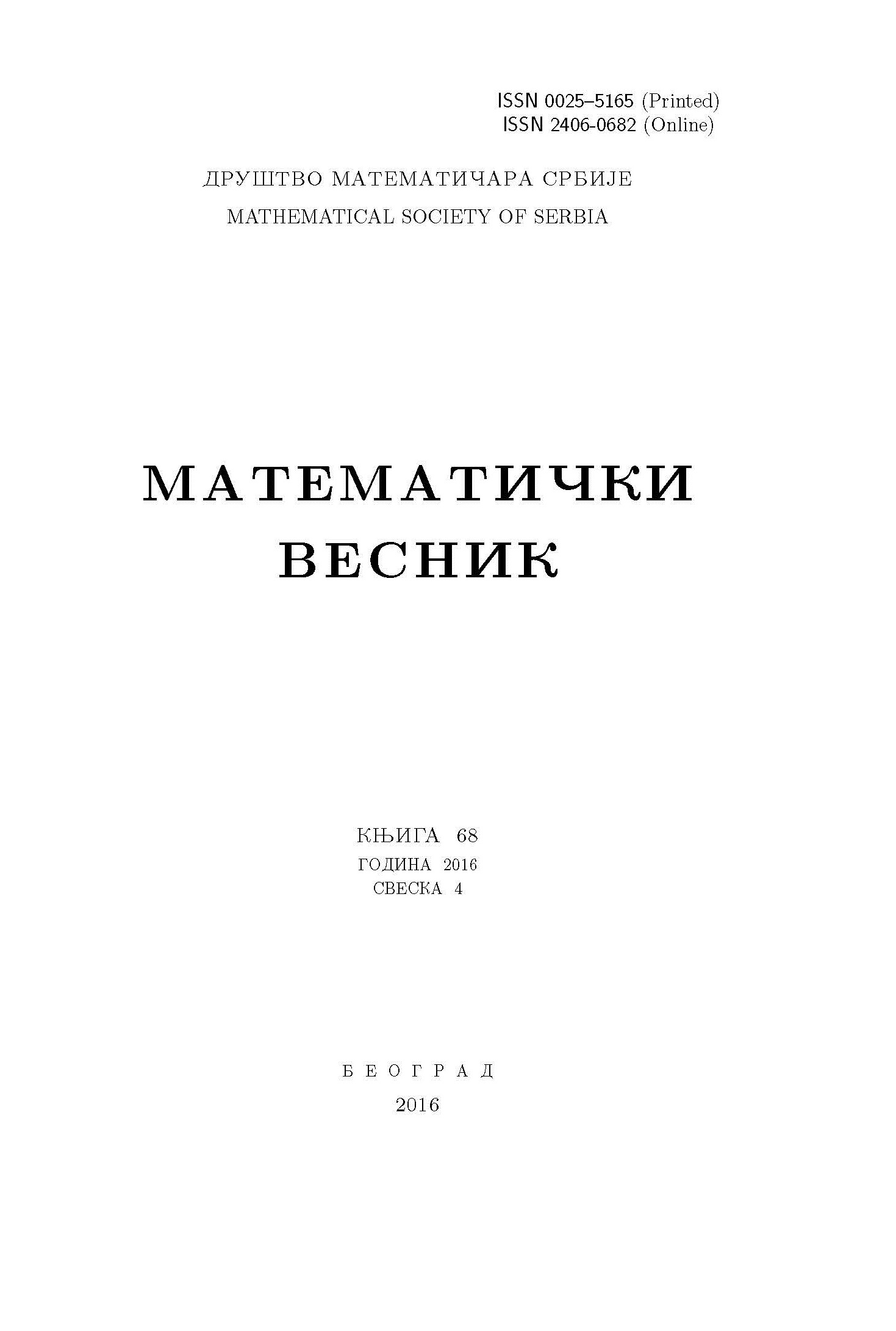﻿ Matematički Vesnik ﻿
 MATEMATIČKI VESNIK МАТЕМАТИЧКИ ВЕСНИКCANTOR SETS AND FIELDS OF REALS G. Kuba AbstractOur main result is a construction of four families ${\cal C}_1,{\cal C}_2,{\cal B}_1,{\cal B}_2$ which are equipollent with the power set of ${\Bbb R}$ and satisfy the following properties. (i) The members of the families are proper subfields $K$ of ${\Bbb R}$ where ${\Bbb R}$ is algebraic over $K$. (ii) Each field in ${\cal C}_1\cup{\cal C}_2$ contains a {\it Cantor set}. (iii) Each field in ${\cal B}_1\cup{\cal B}_2$ is a {\it Bernstein set}. (iv) All fields in ${\cal C}_1\cup{\cal B}_1$ are isomorphic. (v) If $K,L$ are fields in ${\cal C}_2\cup{\cal B}_2$ then $K$ is isomorphic to some subfield of $L$ only in the trivial case $K=L$.Keywords: Transcendental extensions; descriptive set theory. MSC: 12F20, 54H05 Pages:  249$-$259 Volume  74 ,  Issue  4 ,  2022

﻿